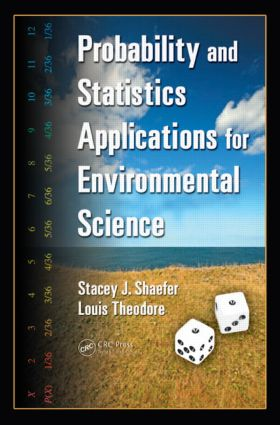# Probability and Statistics Applications for Environmental Science

## 1st Edition

CRC Press

367 pages | 10 B/W Illus.

Hardback: 9780849375613
pub: 2007-02-22
\$150.00
x
eBook (VitalSource) : 9780429124754
pub: 2007-02-22
from \$75.00

FREE Standard Shipping!

### Description

Simple, clear, and to the point, Probability and Statistics Applications for Environmental Science delineates the fundamentals of statistics, imparting a basic understanding of the theory and mechanics of the calculations. User-friendliness, uncomplicated explanations, and coverage of example applications in the environmental field set this book apart from other textbooks on the same subject.

Striking a balance between theory and applied mathematics, the material is divided into three parts. Part I sets the stage with coverage of principles and fundamentals, such as set notation, probability distributions, and the estimation of the mean and variance. Part II discusses traditional statistics applications, centering around the uses of probability distributions, including how they relate to reliability and failure theory. The authors elucidate many of the important distributions, Monte Carlo methods, and fault and event trees. Part III delves into what some have come to define as contemporary statistics. It covers hypothesis testing, Student's t and chi-square tests, regression analysis, analysis of variance (ANOVA), and nonparametric tests.

The book's coverage is thorough, its presentation logical and geared to student's needs. It includes problems and solutions within the text and tables, a glossary of terms, and an introduction to design of experiments in the appendices. The authors, known for their meticulously accurate, articulate, and practical writing style, master the difficult task of explaining very complicated subject matter in a way that is easily understood. The book features a clear, concise presentation that makes both teaching and learning easier.

### Reviews

"This book is the first work that is devoted to the presentation of a practically applicable ecosystems theory. It integrates four aspects of systems ecology, namely, thermodynamics, biochemistry, hierarchical organization and network theory, all of which are illustrated by many examples and exercises to help students better understand the topics."

—Evelyne Bremond-Hoslet, in MAMMALIA, Vol. 77, N° 3 2013

FUNDAMENTALS

PAC. Permutations and Combinations

PDI. Probability Definitions and Interpretations

SNE. Set Notation

BTH. Basic Theorems

COP. Conditional Probability

RAR. Random Variables and Random Numbers

PDF. Introduction to Probability Distributions

EXV. Expected Values

EMV. Estimation of Mean and Variance

BAY. Bayes' Theorem

SPS. Series and Parallel Systems

BIN. The Binomial Distribution

REL. Reliability Relations

HYP. Hypergeometric Distribution

POI. Poisson Distribution

EXP. Exponential Distribution

WBL. Weibull Distribution

NOR. Normal Distribution

LOG. Log-Normal Distribution

MON. Monte Carlo Simulation

FET. Fault Tree and Event Tree Analysis

CONTEMPORARY APPLICATIONS

CIM. Confidence Intervals for Means

CIP. Confidence Intervals for Proportions

HYT. Hypothesis Testing

TMP. Hypothesis Test for Means and Proportions

CHI. Chi-Square Distribution

FFF. The F Distribution

REG. Regression Analysis: Method of Least Squares

NPT. Nonparametric Tests

ANV. Analysis of Variance

Appendix A: Statistics Tables

Appendix B: Glossary of Terms

Appendix C: Introduction to Design of Experiments

Index

Stacey J. Shaefer, Louis Theodore